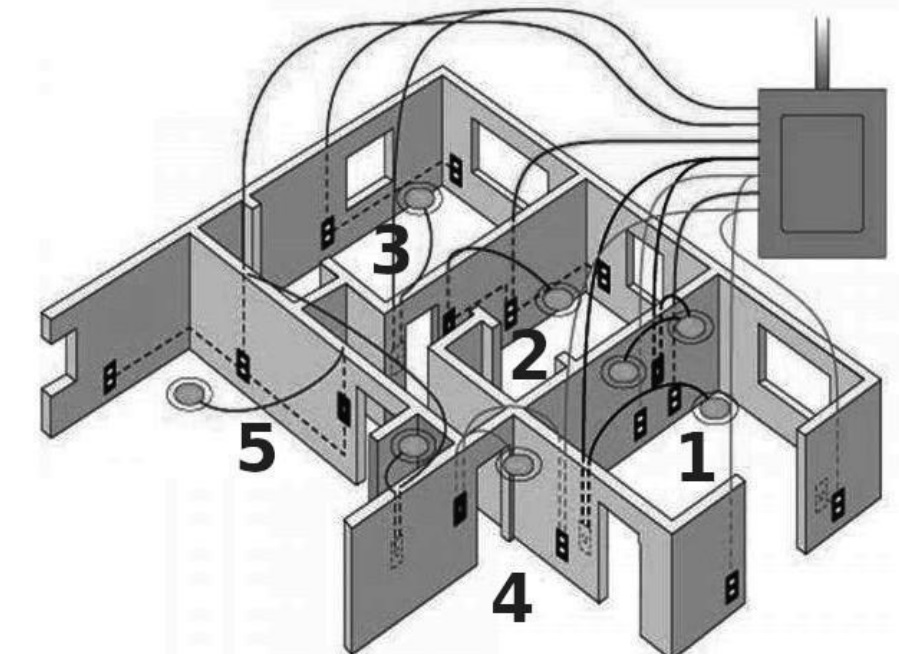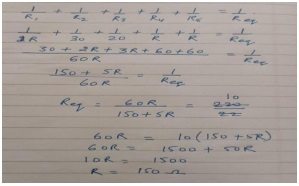CBSE Class 10 Sample Paper Science Solution - For 2023 Boards

Class 10
Solutions to CBSE Sample Paper - Science Class 10

## The diagram above is a schematic diagram of a household circuit. The house shown in the above diagram has 5 usable spaces where electrical connections are made. For this house, the mains have a voltage of 220 V and the net current coming from the mains is 22A.

1. What is the mode of connection to all the spaces in the house from the mains?
2. The spaces 5 and 4 have the same resistance and spaces 3 and 2 have respective resistances of 20Ω and 30Ω. Space 1 has a resistance double that of space 5. What is the net resistance for space 5.
3. What is the current in space 3?
4. What should be placed between the main connection and the rest of the house’s electrical appliances to save them from accidental high electric current?

##googletag.cmd.push(function() { googletag.display('div-gpt-ad-1669298377854-0'); });

(a) All spaces are connected in parallel.

(b) Let Resistance of Space 5 and 4 be R ohms respectively

Resistance of Space 1 = 2 R ohms

Resistance of Space 2 = 30 ohms

Resistance of Space 3 = 20 ohms

Current = 22 A

V= 220 V

Total Resistance= V/I(c) Current in space 3, I 3 can be given by:

I3 = V/R

= 220/ 20

= 11A

(d) Electric Fuses can be used to protect circuits (and individual household appliances) from sudden surges in electric current.

Learn in your speed, with individual attention - Teachoo Maths 1-on-1 Class